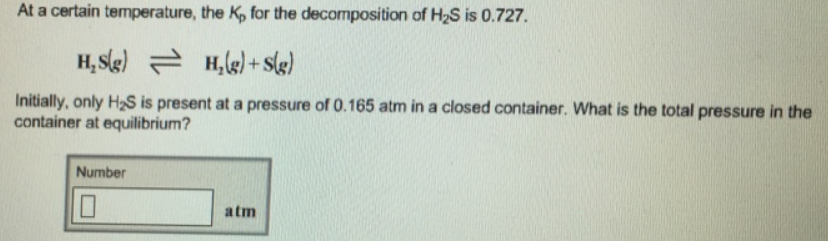# Problem: At a certain temperature, the Kp for the decomposition of H2S is 0.727. H2S (g) ⇌ H2 (g) + S (g) Initially, only H2S is present at a pressure of 0.165 atm in a closed container. What is the total pressure in the equilibrium?

###### FREE Expert Solution
84% (118 ratings)###### Problem Details

At a certain temperature, the Kp for the decomposition of H2S is 0.727.

H2S (g) ⇌ H(g) + S (g)

Initially, only H2S is present at a pressure of 0.165 atm in a closed container. What is the total pressure in the equilibrium?